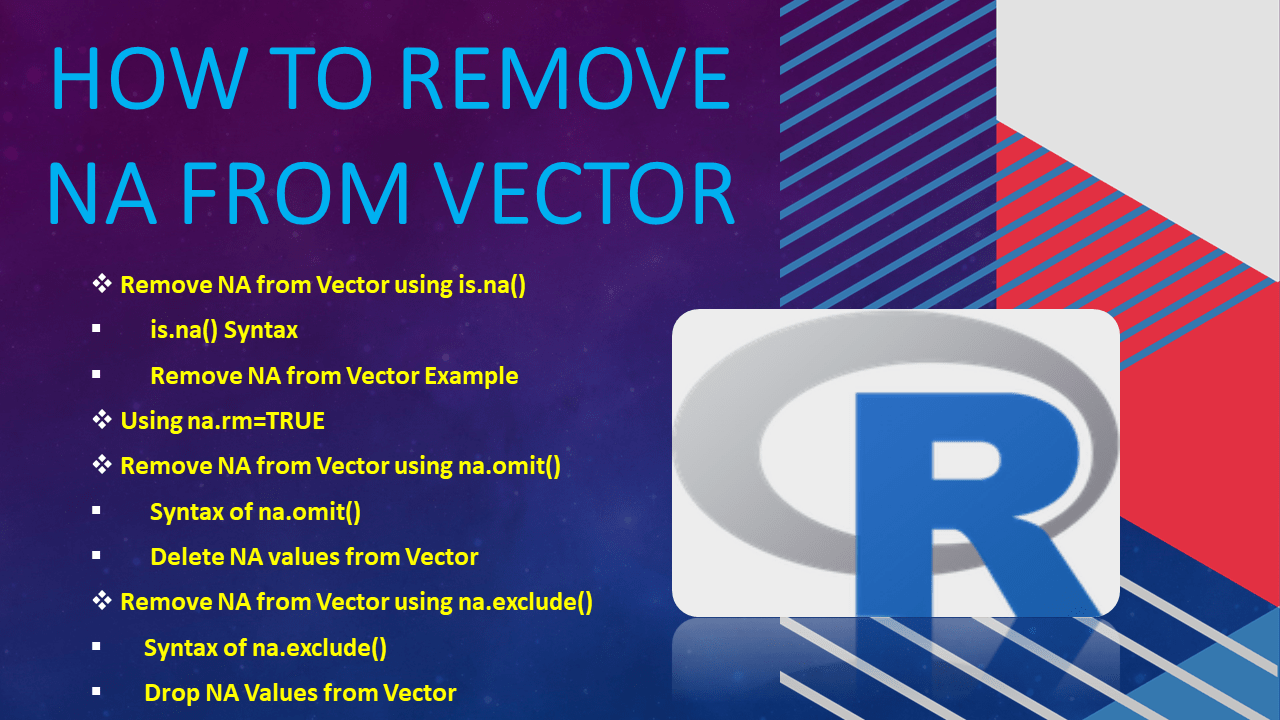# How to Remove NA from Vector?

NA is considered a ‘Missing Values’ or ‘Not Available’ in R and to remove `NA` values from the vector use `na.rm()` or `is.na()` functions. Additionally R also supports several other methods to delete `NA` from the vector.

When you run an arithmetic operation on the R vector that has NA values, you will get results as NA. In general, any operation on an NA becomes a NA. hence, It’s best practice to clean up the vector by removing all NA values before performing an arithmetic operation.

Alternatively, you can also replace NA with 0 before applying any arithmetic or statistical operations.

## 1. Quick Examples of Remove NA from Vector

Following are quick examples of how to remove NA values from R Vector.

``````
# Quick Examples

# Create Vector
ids <- c(10,5,NA,15,10)

# Using is.na()
x <- ids[!is.na(ids)]

# Using na.rm=TRUE
x <- max(ids, na.rm=TRUE)

# Using na.omit()
x <- na.omit(ids)

# Remove NA from string Vector.
states = c('NY','DC',NA,'CA','NV',NA)
x <- na.omit(states)

# Using exclude
x <- na.exclude(states)
print(x)
``````

## 2. Remove NA from Vector using is.na()

The `is.na()` indicates which elements are missing. The `is.na(x)` function returns a logical vector of the same size as `x` with value `TRUE` when the corresponding element in `x` is `NA`.

### 2.1 is.na() Syntax

The following is the syntax of the is.na() function.

``````
# Syntax
vector[!is.na(vector)]
``````

### 2.2 Remove NA from Vector Example

`is.na()` function is used to remove NA values from vector. Actually, is.na() function returns a vector consisting of logical values (i.e. TRUE or FALSE), whereby TRUE indicates a missing value.

``````
# Using is.na()
ids <- c(10,5,NA,15,10)
is.na(ids)

# Output
#  FALSE FALSE  TRUE FALSE FALSE
``````

By negating the result you will get a logical vector with FALSE at the position of NA value. Now use this result on vector bracket notation to remove elements at FALSE position this idally remove NA values from vector.

``````
# Remove NA values from Vector
x <- ids[!is.na(ids)]
print(x)

# Output
#  10  5 15 10
``````

## 3. Using na.rm=TRUE

When used `na.rm=TRUE` with any arithmetic or statistical functions it removes the `NA` values from vector while performing operations. By default `FALSE` is set to `na.rm`.

Following is the syntax of the `na.rm=TRUE` and its usage with `max()` function.

``````
# Syntax of na.rm=TRUE
max(vector, na.rm = TRUE)
``````

Lets use this and calculate sum(), max() and mean() for the Vector. If you don’t remove the NA values from the vector, then all these operations returns NA as a result.

``````
# Using na.rm=TRUE
x <- sum(ids, na.rm=TRUE)
print(x)

x <- max(ids)
print(x)

x <- mean(ids, na.rm=TRUE)
print(x)
``````

## 4. Remove NA from Vector using na.omit()

### 4.1 Syntax of na.omit()

Following is the syntax of the na.omit() function.

``````
# Syntax of na.omit()
na.omit(vector)
``````

### 4.2 Delete NA values from Vector

The following R program removes all NA values from the vector.

``````
# Using na.omit()
x <- na.omit(ids)
print(x)
``````

Yields below output

``````
# Output
 10  5 15 10
attr(,"na.action")
 3
attr(,"class")
 "omit"
``````

## 5. Remove NA from Vector using na.exclude()

### 5.1 Syntax of na.exclude()

Following is the syntax of the na.exclude() function.

``````
# Syntax of na.exclude()
na.exclude(vector)
``````

### 5.2 Drop NA Values from Vector

The following R program removes all NA values from the vector.

``````
# Using exclude
states = c('NY','DC',NA,'CA','NV',NA)
x <- na.exclude(states)
print(x)
``````

Usage and example of na.exclude() function.

``````
# Output
 "NY" "DC" "CA" "NV"
attr(,"na.action")
 3 6
attr(,"class")
 "exclude"
``````

## Conclusion

In this article, you have learned the syntax of is.na(), na.omit() and na.exclude() and how to use these to remove NA values from vector. You can find the complete example from this article at Github R Programming Examples Project.

## References

### Naveen (NNK)

Naveen (NNK) is a Data Engineer with 20+ years of experience in transforming data into actionable insights. Over the years, He has honed his expertise in designing, implementing, and maintaining data pipelines with frameworks like Apache Spark, PySpark, Pandas, R, Hive and Machine Learning. Naveen journey in the field of data engineering has been a continuous learning, innovation, and a strong commitment to data integrity. In this blog, he shares his experiences with the data as he come across. Follow Naveen @ @ LinkedIn